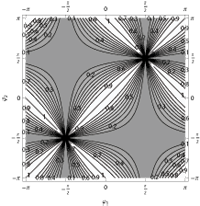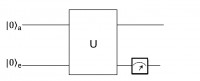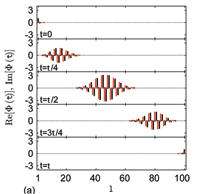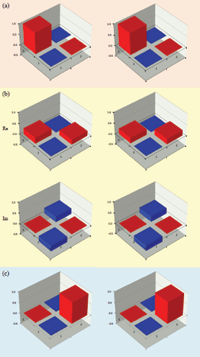June 2011
Mon Tue Wed Thu Fri Sat Sun
« May   Jul »
12345
6789101112
13141516171819
20212223242526
27282930

## Many-Body Effects in a Model of Electromagnetically Induced Transparency

Jose ReslenWe study the effect of inter-band interactions in the absorption profile of a semi-classical model describing electromagnetically induced transparency. We develop a consistent approach using a nonhermitian Hamiltonian to model particle decay. This allow us to characterize the system response for different number of particles so that the effect of particle-interaction on the transmission profile can be studied over a wide range of characteristic parameters.

http://arxiv.org/abs/1106.5240
Quantum Physics (quant-ph)

## Non-Hermitian oscillator Hamiltonians and multiple Charlier polynomials

Hiroshi Miki, Luc Vinet, Alexei Zhedanov

A set of $$r$$ non-Hermitian oscillator Hamiltonians in $$r$$ dimensions is shown to be simultaneously diagonalizable. Their spectra is real and the common eigenstates are expressed in terms of multiple Charlier polynomials. An algebraic interpretation of these polynomials is thus achieved and the model is used to derive some of their properties.

http://arxiv.org/abs/1106.5243
Mathematical Physics (math-ph)

## Galois Conjugates of Topological Phases

Michael H. Freedman, Jan Gukelberger, Matthew B. Hastings, Simon Trebst, Matthias Troyer, Zhenghan WangGalois conjugation relates unitary conformal field theories (CFTs) and topological quantum field theories (TQFTs) to their non-unitary counterparts. Here we investigate Galois conjugates of quantum double models, such as the Levin-Wen model. While these Galois conjugated Hamiltonians are typically non-Hermitian, we find that their ground state wave functions still obey a generalized version of the usual code property (local operators do not act on the ground state manifold) and hence enjoy a generalized topological protection. The key question addressed in this paper is whether such non-unitary topological phases can also appear as the ground states of Hermitian Hamiltonians. Specific attempts at constructing Hermitian Hamiltonians with these ground states lead to a loss of the code property and topological protection of the degenerate ground states. Beyond this we rigorously prove that no local change of basis can transform the ground states of the Galois conjugated doubled Fibonacci theory into the ground states of a topological model whose Hermitian Hamiltonian satisfies Lieb-Robinson bounds. These include all gapped local or quasi-local Hamiltonians. A similar statement holds for many other non-unitary TQFTs. One consequence is that the “Gaffnian” wave function cannot be the ground state of a gapped fractional quantum Hall state.

http://arxiv.org/abs/1106.3267
Strongly Correlated Electrons (cond-mat.str-el); Mesoscale and Nanoscale Physics (cond-mat.mes-hall); Mathematical Physics (math-ph)

## Entanglement Efficiencies in PT-Symmetric Quantum Mechanics

Christian Zielinski, Qing-hai WangThe degree of entanglement is determined for arbitrary states of a PT-symmetric bipartite composite system. We characterize the rate with which entangled states are generated and show that this rate can be quantified by a small set of parameters. These relations allow one in principle to increase the efficiency of these systems to entangle states. It is also noticed that many relations resemble corresponding ones in conventional quantum mechanics.

http://arxiv.org/abs/1106.3856
High Energy Physics – Theory (hep-th); Quantum Physics (quant-ph)

## Shortcuts to adiabaticity for non-Hermitian systems

S. Ibáñez, S. Martí nez-Garaot, Xi Chen, E. Torrontegui, J. G. MugaAdiabatic processes driven by non-Hermitian, time-dependent Hamiltonians may be sped up by generalizing inverse engineering techniques based on Berry’s transitionless driving algorithm or on dynamical invariants. We work out the basic theory and examples described by two-level Hamiltonians: the acceleration of rapid adiabatic passage with a decaying excited level and of the dynamics of a classical particle on an expanding harmonic oscillator.

http://arxiv.org/abs/1106.2776
Quantum Physics (quant-ph)

## Time-Reversal Symmetry in Non-Hermitian Systems

Masatoshi Sato, Kazuki Hasebe, Kenta Esaki, Mahito Kohmoto

For ordinary hermitian Hamiltonians, the states show the Kramers degeneracy when the system has a half-odd-integer spin and the time reversal operator obeys $$\Theta^2=-1$$, but no such a degeneracy exists when $$\Theta^2=+1$$. Here we point out that for non-hermitian systems, there exists a degeneracy similar to Kramers even when $$\Theta^2=+1$$. It is found that the new degeneracy follows from the mathematical structure of split-quaternion, instead of quaternion from which the Kramers degeneracy follows in the usual hermitian cases. Furthermore, we also show that particle/hole symmetry gives rise to a pair of states with opposite energies on the basis of the split quaternion in a class of non-hermitian Hamiltonians. As concrete examples, we examine in detail NxN Hamiltonians with N=2 and 4 which are non-hermitian generalizations of spin 1/2 Hamiltonian and quadrupole Hamiltonian of spin 3/2, respectively.

http://arxiv.org/abs/1106.1806
Statistical Mechanics (cond-mat.stat-mech); Mathematical Physics (math-ph); Quantum Physics (quant-ph)

## Reply on Comments on “Observation of a Fast Evolution in a Parity-time-symmetric System”(ArXiv:1106.1550)

Chao Zheng, Liang Hao, Gui Lu Long

Masillo  commented on our manuscript  “Observation of a Fast Evolution in a Parity-time-symmetric System”, pointing out a contradiction of our work with Ref.. In this reply, we pointed out there is no disagreement between Masillo’s comment and our work in Ref. . The efficiency cost pointed out in Ref.\cite{masillo} exists, namely to obtain the PT-symmetric hamiltonian evolution, one has to make a measurement on the auxiliary qubit and the auxiliary qubit is at state $$\left|0\right \rangle$$ only probabilistically. This is reflected in the amplitude of the spectrum in the NMR quantum simulation. As a result, we made a small modification in a new version of the Ref. , and Fig. 2 of Ref. has been replaced by spectra of two different $$\alpha$$’s in order to illustrate this fact.

http://arxiv.org/abs/1106.1848
Quantum Physics (quant-ph)

## Comments on “Observation of Fast Evolution in Parity-Time-Symmetric System”

Fabio MasilloIn the paper “Observation of Fast Evolution in Parity-Time-Symmetric System” the authors propose a physical apparatus for the realization of a faster than Hermitian evolution. This last appears in contrast with the conclusions obtained in our paper “Some Remarks on Quantum Brachistochrone”. We will clarify this apparent contradiction and some problematic aspects of the treatment in .

http://arxiv.org/abs/1106.1550
Quantum Physics (quant-ph)

## Perfect State Transfer in PT-symmetric Non-Hermitian Networks

X. Z. Zhang, L. Jin, Z. SongWe systematically study the parity- and time-reversal- (PT) symmetric non-Hermitian version of a quantum network proposed in the work of Christandl et al. [Phys. Rev. Lett. 92, 187902 (2004)]. We show that such an extended model still allows conditional perfect state transfer within the unbroken PT-symmetric region, but not arbitrary. This is due to the fact that the evolution operator at certain period is equivalent to the PT operator for the real-valued wavefunction in the elaborate PT-symmetric Hilbert space. The critical behavior of the eigenstates is also discussed at the exceptional point.

http://arxiv.org/abs/1106.0087
Quantum Physics (quant-ph)

## Observation of Fast Evolution in Parity-Time-Symmetric System

Chao Zheng, Liang Hao, Gui Lu LongTo find and realize the optimal evolution between two states is significant both in theory and application. In quantum mechanics, the minimal evolution is bounded by the gap between the largest and smallest eigenvalue of the Hamiltonian. In the parity-time-symmetric(PT-symmetric) Hamiltonian theory, it was predicted that the optimized evolution time can be reduced drastically comparing to the bound in the Hermitian case, and can become even zero. In this Letter, we report the experimental observation of the fast evolution of a PT-symmetric Hamiltonian in an nuclear magnetic resonance (NMR) quantum system. The experimental results demonstrate that the PT-symmetric Hamiltonian can indeed evolve much faster than that in a quantum system, and time it takes can be arbitrary close to zero.

http://arxiv.org/abs/1105.6157
Quantum Physics (quant-ph)